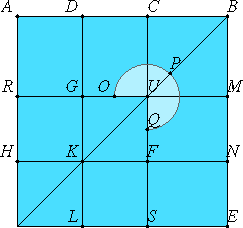# Proposition 3

If a straight line is cut in extreme and mean ratio, then the square on the sum of the lesser segment and the half of the greater segment is five times the square on the half of the greater segment.

Cut any straight line AB in extreme and mean ratio at the point C, and let AC be the greater segment. Bisect AC at D.

I say that the square on BD is five times the square on DC.I.46

Describe the square AE on AB, and draw the figure.

Since AC is double DC, therefore the square on AC is quadruple the square on DC, that is, RS is quadruple FG.

And, since the rectangle AB by BC equals the square on AC, and CE is the rectangle AB by BC, therefore CE equals RS.

Again, since AD equals DC, therefore HK also equals KF.

Hence the square GF equals the square HL.

Therefore GK equals KL, that is MN equals NE, hence MF equals FE.

But MF equals CG, therefore CG equals FE.

Add CN to each, therefore the gnomon OPQ equals CE.

But CE was proved quadruple GF, therefore the gnomon OPQ is also quadruple the square FG. Therefore the sum of the gnomon OPQ and the square FG is five times FG.

But the sum of the gnomon OPQ and the square FG is the square DN. And DN is the square on DB, and GF is the square on DC. Therefore the square on DB is five times the square on DC.

Therefore, if a straight line is cut in extreme and mean ratio, then the square on the sum of the lesser segment and the half of the greater segment is five times the square on the half of the greater segment.

Q.E.D.

## Guide

#### Use of this proposition

This result is needed in proposition XIII.16 to show the icosahedron is inscribed in the given sphere.